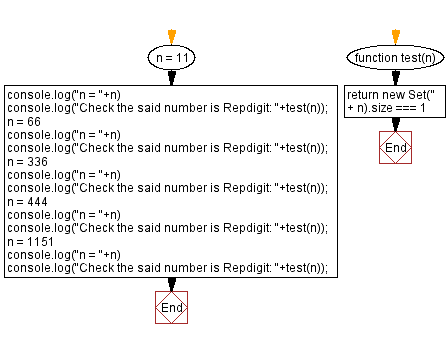# JavaScript: Check an integer is Repdigit number or not

## JavaScript Math: Exercise-80 with Solution

Write a JavaScript program to check whether a given integer is a Repdigit or not.

From Wikipedia -
In recreational mathematics, a repdigit or sometimes monodigit is a natural number composed of repeated instances of the same digit in a positional number system (often implicitly decimal). The word is a portmanteau of repeated and digit. Examples are 11, 666, 4444, and 999999.

Test Data:
(11) -> true
(66) -> true
(336) -> false
(444) -> true
(1151) -> false

Sample Solution:

HTML Code:

``````<!DOCTYPE html>
<html>
<meta charset="utf-8">
<title>JavaScript program to check an integer is Repdigit number or not</title>
<body>

</body>
</html>
```
```

JavaScript Code:

``````function test(n) {
return new Set('' + n).size === 1
}

n = 11
console.log("n = "+n)
console.log("Check the said number is Repdigit: "+test(n));
n = 66
console.log("n = "+n)
console.log("Check the said number is Repdigit: "+test(n));
n = 336
console.log("n = "+n)
console.log("Check the said number is Repdigit: "+test(n));
n = 444
console.log("n = "+n)
console.log("Check the said number is Repdigit: "+test(n));
n = 1151
console.log("n = "+n)
console.log("Check the said number is Repdigit: "+test(n));
```
```

Sample Output:

```n = 11
Check the said number is Repdigit: true
n = 66
Check the said number is Repdigit: true
n = 336
Check the said number is Repdigit: false
n = 444
Check the said number is Repdigit: true
n = 1151
Check the said number is Repdigit: false
```

Flowchart:Live Demo:

See the Pen javascript-math-exercise-80 by w3resource (@w3resource) on CodePen.

Improve this sample solution and post your code through Disqus

What is the difficulty level of this exercise?

Test your Programming skills with w3resource's quiz.

﻿